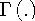Functions and CALL Routines

# LOGBETA Function

Returns the logarithm of the beta function.
 Category: Mathematical

## Syntax

 LOGBETA(a,b)

### Arguments

a

is the first shape parameter, where a>0.

b

is the second shape parameter, where b>0.

The LOGBETA function is mathematically given by the equationwhereis the gamma function.

If the expression cannot be computed, LOGBETA returns a missing value.

SAS Statements Results
`LOGBETA(5,3);`
`-4.653960350`

Function:Previous Page | Next Page | Top of Page